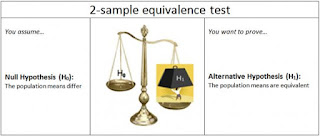## Monday, November 06, 2017

### Statistical test for "no difference"

To most researchers and practitioners using statistical inference, the popular hypothesis testing universe consists of two hypotheses:
H0 is the null hypothesis of "zero effect"
H1 is the alternative hypothesis of "a non-zero effect"

The alternative hypothesis (H1) is typically what the researcher is trying to find: a different outcome for a treatment and control group in an experiment, a regression coefficient that is non-zero, etc. Recently, several independent colleagues have asked me if there's a statistical way to show that an effect is zero, or, that there's no difference between groups. Can we simply use the above setup? The answer is no. Can we simply reverse the hypotheses? Uh-uh, because the "equal" must be in H0.Minitab has equivalence testing (from http://blog.minitab.com/blog/statistics-and-quality-data-analysis/equivalence-testing-for-quality-analysis-part-i-what-are-you-trying-to-prove)
Here's why: In the classic setup the hypotheses are stated about the population of interest, and we take a sample from that population to test them. In this non-symmetrical setup, H0 is assumed to be true unless otherwise proven by the result in the sample. Hypothesis testing has its roots in Karl Popper's falsifiability principle, where any claim about the existence of an effect cannot be made unless it is first shown that a situation of no effect is untenable. This is similar to a democratic justice system, where the defendant is assumed not-guilty unless proven so. The burden of proof lies on the researcher/data. That's why we reject H0 with sufficient evidence or not reject H0, if we don't have sufficient evidence against it. This setup is not designed to arrive at a conclusion that H0 is true.

In a 2013 Letter to the Editor of Journal of Sports Sciences, titled Testing the null hypothesis: the forgotten legacy of Karl Popper? Mick Wilkinson suggests that this setup is the opposite of what a researcher should be doing according to the scientific method, and in fact "Our work should remain driven by conjecture and attempted falsification such that it is always the null hypothesis that is tested. The write up of our studies should make it clear that we are indeed testing the null hypothesis and conforming to the established and accepted philosophical conventions of the scientific method." He therefore suggests the following sequence:

1. null hypothesis tests are carried out to first establish that a population effect is in fact unlikely to be zero
2. a confidence-interval based approach estimates what the magnitude of effect might plausibly be
3. a probability associated with the likelihood of the population effect exceeding an apriori smallest meaningful effect is calculatedWhile this provides a relevant criticism of the hypothesis testing paradigm, it does not directly provide a test of equivalence! The good news is that equivalence testing is a popular such scenario in pharmacokinetics, arising, for example, when a pharmaceutical wants to show that their developed generic drug is equivalent to a brand drug. This is termed bioequivalence. In other words, H1 is "the drugs are equivalent". The approach used there is the following:

1. set up an equivalence bound that determines the smallest clinically-meaningful effect size of interest
2. calculate a confidence interval around the observed effect size (say, difference between the mean outcomes of the generic and brand drugs)
3. if the confidence interval includes the equivalence bound, then the groups are equivalent; otherwise they are not equivalent
The Wikipedia article on Equivalence Test points out two additional interesting uses of equivalence testing:
• Avoiding misinterpretation of large p-values in ordinary testing as evidence for H0: "Equivalence tests can be performed in addition to null-hypothesis significance tests. This might prevent common misinterpretations of p-values larger than the alpha level as support for the absence of a true effect."
• The confidence interval used in equivalence testing can help distinguish between statistical significance and practical/clinical significance: if it includes/excludes the value 0, that indicates statistical insignificance/significance between the groups (or an effect), while if it includes/excludes the equivalence bound that indicates practical insignificance/significance. The four options are shown in the figure.Statistical vs. practical significance (from https://en.wikipedia.org/wiki/Equivalence_test)
How will sample size affect equivalence testing? We know that in ordinary hypothesis testing a sufficiently large sample will lead to detecting practically insignificant effects by generating a very small p-value, which is bad news for those relying on classic hypothesis testing! My colleague Foster Provost from NYU once challenged me how I could trust a statistical method that breaks down with large samples - a poignant thought that eventually led to my co-authored paper Too Big To Fail: Large Samples and the p-value Problem  (Lin et al., ISR 2013). What about equivalence tests? In equivalence testing, a very large sample will behave properly: with more data we'll get narrower confidence intervals (more certainty). A practically insignificant difference will therefore generate a narrow confidence interval that excludes the equivalence boundary (=equivalence). In contrast, a practically significant difference will generate a narrow confidence interval that completely exceeds the equivalence boundary (non-equivalence).